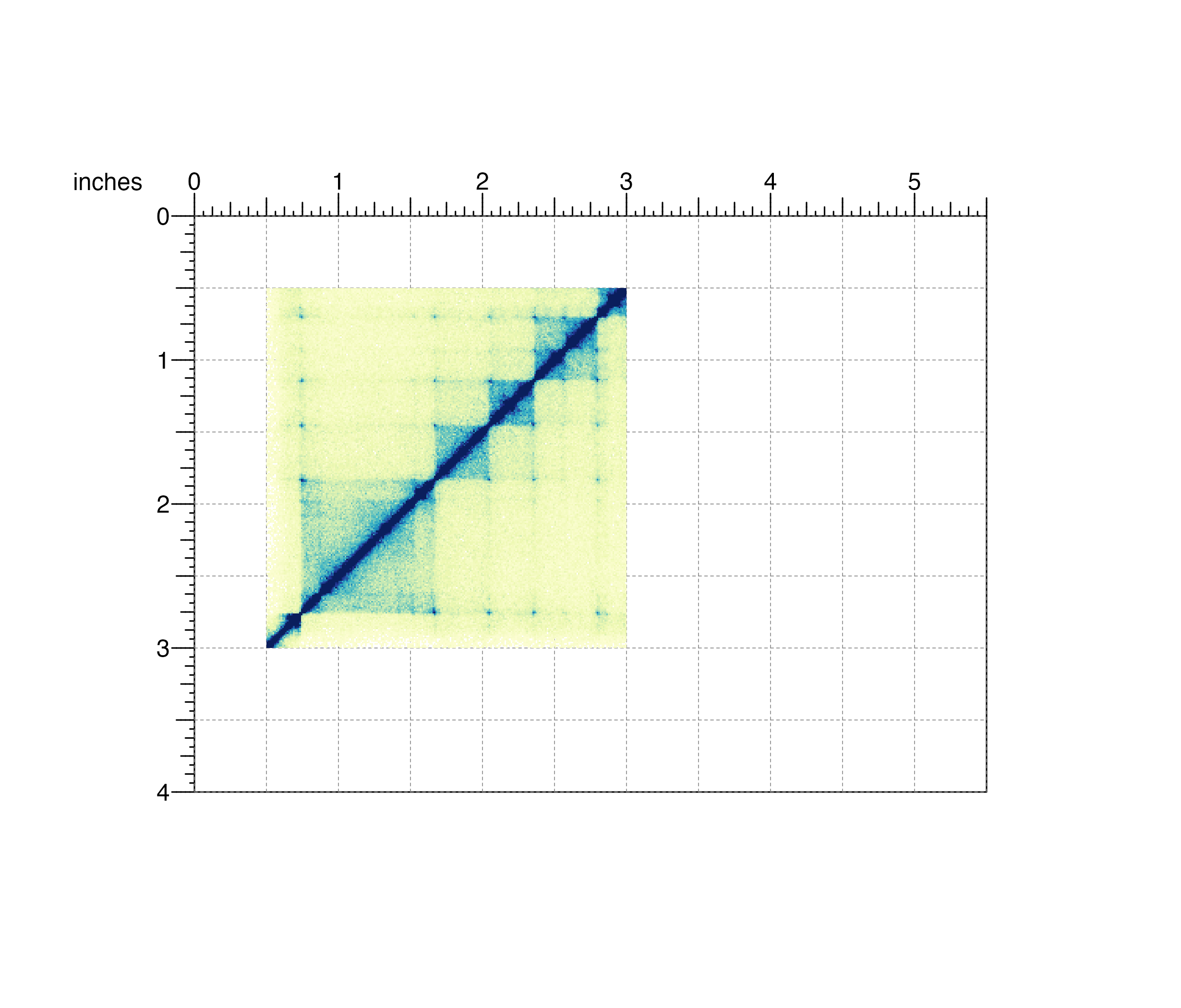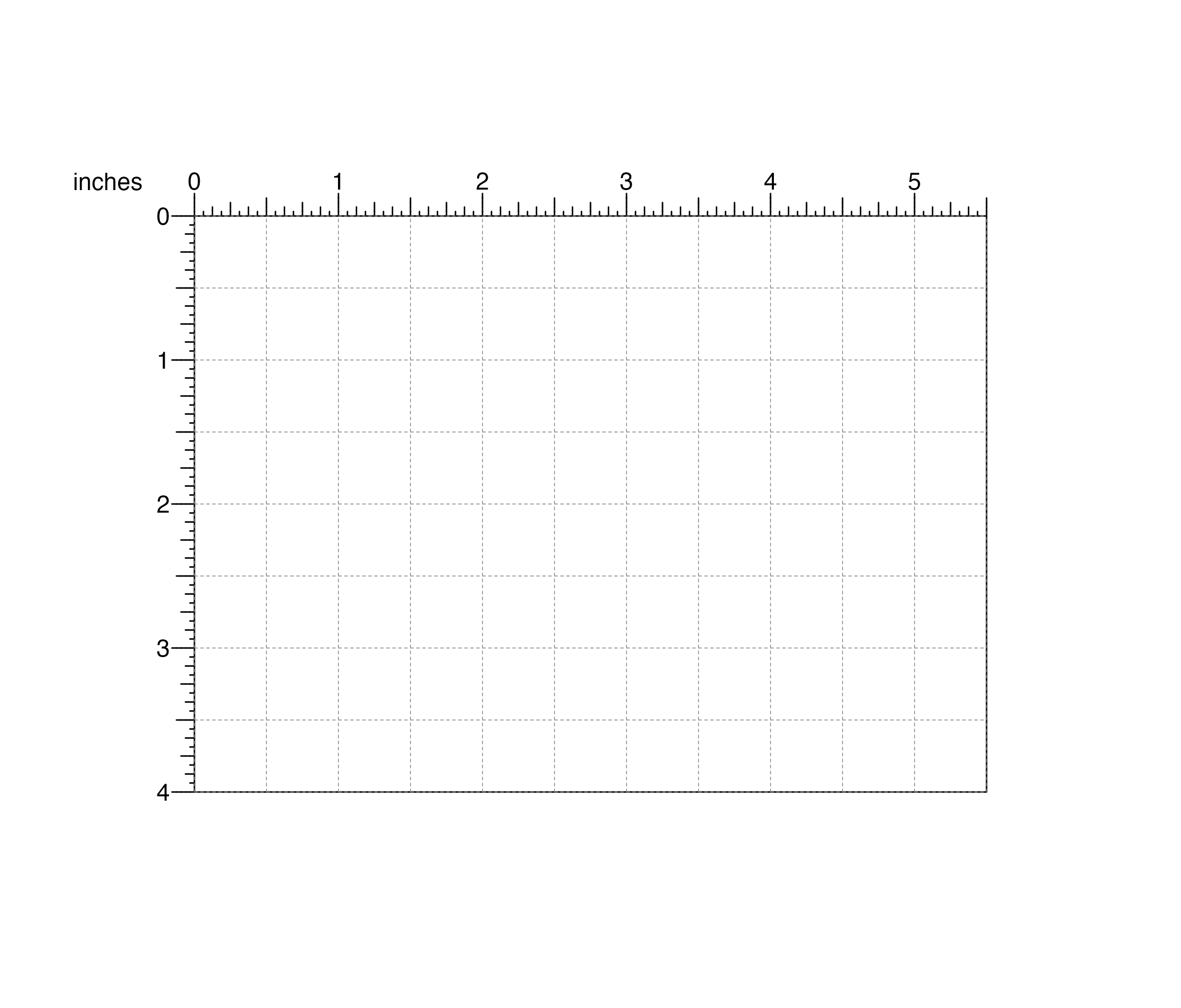Remove plotgardener plots and annotations

pagePlotRemove(plot)

## Arguments

plot

Plot object to be removed, defined by the output of a plotgardener plotting function.

None.

## Examples

## Load Hi-C data
library(plotgardenerData)
data("IMR90_HiC_10kb")

## Create page
pageCreate(width = 5.5, height = 4, default.units = "inches")

## Plot and place a square Hi-C plot
hicPlot <- plotHicSquare(
data = IMR90_HiC_10kb, resolution = 10000,
zrange = c(0, 70),
chrom = "chr21",
chromstart = 28000000, chromend = 30300000,
x = 0.5, y = 0.5, width = 2.5, height = 2.5,
just = c("left", "top"),
default.units = "inches"
)
#> Read in dataframe.  Assuming 'chrom' in column1 and 'altchrom' in column2. 10000 BP resolution detected.
#> hicSquare[hicSquare1]## Remove square Hi-C plot from page
pagePlotRemove(plot = hicPlot)